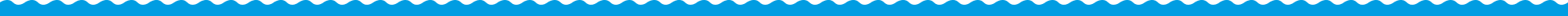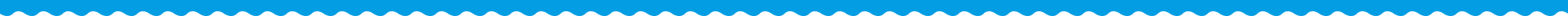# Online High School Tutoring

The 1300HOMETUTOR team have been supporting high school students Australia-wide for over a decade. We understand that most students will experience stress and anxiety at some point in time during high school, and for some, this can impact their lives significantly. With this in mind, we have developed a comprehensive online tutoring service that allows Year 7 to Year 12 students to achieve success whilst also being able to enjoy their time at high school.

Our students have access to an extensive range of proven lesssons that follow the Australian Curriculum and they also have the option to access daily support in the forms of English Tutoring and Maths Tutoring, which we deliver via telephone, Skype and Facetime.

The 1300 HOMETUTOR benefits:
• School Assignment Support
• Daily Homework Support
• Student Ability Assessments
• Reward Certificates
• Daily Parent's Report
• Structured Individualised Lessons
WATCH OUR VIDEO TO FIND OUT MORE

## High School Maths - Lesson Structure

### Year 7 Maths Lessons

Chapter 1: Number
• Whole numbers
• Integer
• Powers
• Fractions and Percentages
• Decimals
• Rates, Ratio and Proportion
• Four operations
Chapter 2: Measurement
• Perimeter
• Area
• Mass
• Times
• Temperature
Chapter 3: Space
• Lines
• Circles
• Angle Sum of a polygon
• Symmetry
• 3D objects
Chapter 4: Algebra
• Arithmetic laws
• Ordered pairs
• Order of operations
• Expressions and rules
• Equations
Chapter 5: Chance and Data
• Theoretical probability
• Surveys
• Graphs and tables
• Average

### Year 8 Maths Lessons

Chapter 1: Number
• Rational, Irrational numbers
• Indices
• Square roots
• Percentages
• Rates
Chapter 2: Measurement
• Area
• Area of circles
• Volume
Chapter 3: Space
• 3D
• Angles
• External angles
• Congruence
• Similar shapes
• Constructions
Chapter 4: Algebra
• Equations
• Algebraic expressions
• Ordered pairs
• Variables
Chapter 5: Chance and Data
• Probability
• Grouped data

### Year 9 Maths Lessons

Chapter 1: Number
• Indices
• Significant figure
• Standard form
• Interest
• GST
Chapter 2: Measurement
• Circumference
• Volume
• Capacity
Chapter 3: Space
• Angles properties
• Tessellations
• Line Graphs
• Bearings
Chapter 4: Algebra
• Symbol
• Algebraic expressions
• Substitution
• Expanding
• Factorizing
• Equations
• Simultaneous equations
Chapter 5: Chance and Data
• Data Display
• Venn Diagrams

### Year 10 Maths Lessons

Chapter 1: Number
• Fractional Indices
• Irrational Numbers
• Rationalisation
• Recurring Decimals
Chapter 2: Measurement
• Factorising
• Inequalities
• Simultaneous Equations
Chapter 3: Space
• Pythagoras
• Special Triangles
• Ratios
• Inverse Rations
Chapter 4: Algebra
• Coordinate Geometry
• Lines
• Parabolas
• Loci
• Circles
• Surface Areas
Chapter 5: Chance and Data
• Standard Deviation
• Mutually Exclusive Events
• Independent Events

### Year 11 Maths Lessons

Chapter 1: Algebra
• Function
• Factoring
• Rearrangement
Chapter 2: Index
• Surds
• Logarithms
Chapter 3: Sequence
• Recursive definition
• Sigma
• Arithmetic
• Geometric
• Growth and decay
Chapter 4: Graphs
• Circle graph
• Cubic graph
• Square root graph
Chapter 5: Trigonometry
• Trigonometric graphs
• Sine rule
• Cosine rule
Chapter 6: Differentiation
• Limit
• Derivatives of polynomials
• Tangents
• Normals
• Stationary Points
Chapter 7: Integration
• Anti-derivatives
• Evaluating the constant

### Year 12 Maths Lessons

Chapter 1: Algebra
• Remainder,factor theorem
• Inequalities
• Sum and Product of roots
Chapter 2: Function
• Domain and range
• Even,Odd, periodic functions
• Composite functions
• Inverse functions
Chapter 3: Trigonometry
• Graph
• The reciprocal trig functions
• Identities
• Formulas
• Composition
• Inverse
• Equations
• Applications
Chapter 4: Graph
• Hyperbola graph
• Absolute value graph
• Exponential graph, log graph
• Intersection of lines and curves
Chapter 5: Complex number
• Complex numbers
• Calculation of complexe numbers
• Polar form
• De-moivre`s theorem
• Complex roots
Chapter 6: Differentiation
• Limit
• Derived functions
• Differentiation of Polynomial
• Differentiation of functions
• Implicit Differentiation
• Stationary points
• Application of Differentiation
Chapter 7: Integration
• Integration of functions
• Definite integrals
• Area
• Volume
• Numerical Integration
Chapter 8: Differential Equation
• Family of solutions
• Applications
Chapter 9: Vector
• Vectors
• Scalar product#### SEND A QUICK ENQUIRY

Please tick to verify that you are not a robot#### Testimonials

We have noticed a huge difference since using
1300 Home Tutor